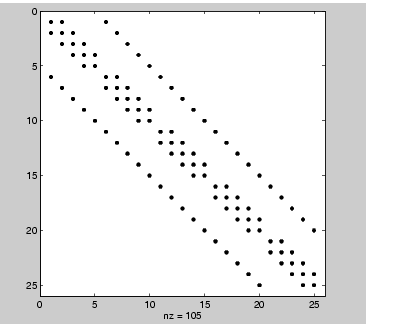MATLAB Function Referencekron

Kronecker tensor product

Syntax

• ```K =` `kron(X,Y)
```

Description

```K = kron(X,Y) ``` returns the Kronecker tensor product of `X` and `Y`. The result is a large array formed by taking all possible products between the elements of `X` and those of `Y`. If `X` is `m`-by-`n` and `Y` is `p`-by-`q`, then `kron(X,Y)` is `m*p`-by-`n*q`.

Examples

If `X` is 2-by-3, then `kron(X,Y)` is

• ```[` `X(1,1)*Y` `X(1,2)*Y` `X(1,3)*Y
`  `X(2,1)*Y` `X(2,2)*Y` `X(2,3)*Y` `]
```

The matrix representation of the discrete Laplacian operator on a two-dimensional, `n`-by-`n` grid is a `n^2`-by-`n^2` sparse matrix. There are at most five nonzero elements in each row or column. The matrix can be generated as the Kronecker product of one-dimensional difference operators with these statements:

• ```` `I` `=` `speye(n,n);
` `E` `=` `sparse(2:n,1:n-1,1,n,n);
` `D` `=` `E+E'-2*I;
` `A` `=` `kron(D,I)+kron(I,D);
```

Plotting this with the `spy` function for `n = 5` yields:© 1994-2005 The MathWorks, Inc.